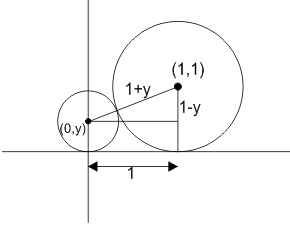#### Let C be the circle with centre at (1, 1) and radius = 1. If T is the circle centred at (0, y), passing through origin and touching the circle C externally, then the radius of T is equal to : Option 1)Option 2)Option 3)Option 4)As we learnt in

Circle touching y-axis and having radius r -- wherein

Where g is a variable parameter.According to Pythagorus theorem,Option 1)This option is incorrect.

Option 2)This option is correct.

Option 3)This option is incorrect.

Option 4)This option is incorrect.

##### Posted by